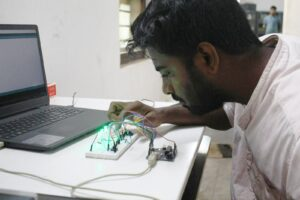# LOGIC GATES USING ARDUINO UNO

A basic gate is defined as a component with one or more inputs and one output.  The inputs and outputs are all digital.

There are three fundamental gates and a total of seven basic logic gates (plus several derivatives). The gate will set its output to either zero or one, based on the state of the input signals.  It uses the rules of Boolean algebra to determine the output condition. The relationship between the input and output logic levels on a gate can be best illustrated using what is known as a truth table.

Simulating Gates with an Arduino:

All of the Boolean algebra functions performed by the basic logic gates can also be emulated on an Arduino. With that in mind, a logic gate emulator that will emulate six of the seven basic gates has been constructed. It doesn’t include the NOT gate because it only has one input whereas the other gates have two.

Project on logic gates using Arduino Uno board consists of 8 LEDs and 8 resistors for the LEDs and two resistors for the pushbutton switches. The LEDs are connected for the logical output and the pushbutton switches are used for the logical input. The positive terminals of the LEDs are connected to the respective terminals of the Arduino board. The negative terminals are connected to the ground of the Arduino.

The green LEDs are for the logic outputs and the red LEDs are for the input.

The dropping resistors for the LEDs are all 220 ohms.

The two pulldown resistors for the pushbutton switches are 2.2k each.

First, define some Boolean variables to represent the logic states of both the two inputs and six outputs. Next, define some integers to represent the connections to the Arduino from the LEDs and push buttons.

It displays logic states on the serial monitor, so in the Setup routine, initialize the monitor at 9600 bps. The rest of the Setup is used to define the LEDs as outputs and pushbuttons as inputs.

In the Loop, it starts by reading the state of the two pushbuttons, and then displaying the results on the two Red LEDs marked “A” and “B”.

The actual Boolean math consists of the following four characters:

• NOT =!
• AND = &
• OR = |
• XOR = ^

We use the NOT function (!) to create the NOR, NAND, and XNOR gates.

After determining the Boolean results they are sent to both the serial monitor and the LEDs.  Following a short delay the whole Loop repeats.

Load the sketch and give it a try. Cycle through all four combinations of the two pushbuttons and observe the LED statuses, as well as the status on the serial monitor.

This would be a great training tool for testing our knowledge of digital logic.

Arduino gates using code